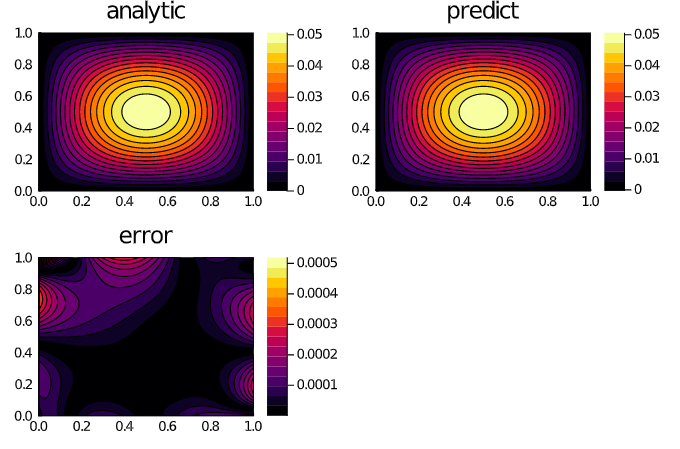## NeuralNetDiffEq.jl

Physics-Informed Neural Networks (PINN) and Deep BSDE Solvers of Differential Equations for Scientific Machine Learning (SciML) accelerated simulation
Popularity
755 Stars
Updated Last
5 Months Ago
Started In
March 2017

# NeuralPDE

NeuralPDE.jl is a solver package which consists of neural network solvers for partial differential equations using physics-informed neural networks (PINNs). This package utilizes neural stochastic differential equations to solve PDEs at a greatly increased generality compared with classical methods.

## Installation

Assuming that you already have Julia correctly installed, it suffices to install NeuralPDE.jl in the standard way, that is, by typing `] add NeuralPDE`. Note: to exit the Pkg REPL-mode, just press Backspace or Ctrl + C.

## Tutorials and Documentation

For information on using the package, see the stable documentation. Use the in-development documentation for the version of the documentation, which contains the unreleased features.

## Features

• Physics-Informed Neural Networks for ODE, SDE, RODE, and PDE solving
• Ability to define extra loss functions to mix xDE solving with data fitting (scientific machine learning)
• Automated construction of Physics-Informed loss functions from a high level symbolic interface
• Integrated logging suite for handling connections to TensorBoard
• Handling of (partial) integro-differential equations and various stochastic equations
• Specialized forms for solving `ODEProblem`s with neural networks
• Compatability with Flux.jl and Lux.jl for all of the GPU-powered machine learning layers available from those libraries.
• Compatability with NeuralOperators.jl for mixing DeepONets and other neural operators (Fourier Neural Operators, Graph Neural Operators, etc.) with physics-informed loss functions

## Example: Solving 2D Poisson Equation via Physics-Informed Neural Networks

```using NeuralPDE, Lux, ModelingToolkit, Optimization, OptimizationOptimisers
import ModelingToolkit: Interval, infimum, supremum

@parameters x y
@variables u(..)
Dxx = Differential(x)^2
Dyy = Differential(y)^2

# 2D PDE
eq = Dxx(u(x, y)) + Dyy(u(x, y)) ~ -sin(pi * x) * sin(pi * y)

# Boundary conditions
bcs = [u(0, y) ~ 0.0, u(1, y) ~ 0,
u(x, 0) ~ 0.0, u(x, 1) ~ 0]
# Space and time domains
domains = [x ∈ Interval(0.0, 1.0),
y ∈ Interval(0.0, 1.0)]
# Discretization
dx = 0.1

# Neural network
dim = 2 # number of dimensions
chain = Lux.Chain(Dense(dim, 16, Lux.σ), Dense(16, 16, Lux.σ), Dense(16, 1))

@named pde_system = PDESystem(eq, bcs, domains, [x, y], [u(x, y)])
prob = discretize(pde_system, discretization)

callback = function (p, l)
println("Current loss is: \$l")
return false
end

res = Optimization.solve(prob, ADAM(0.1); callback = callback, maxiters = 4000)
prob = remake(prob, u0 = res.minimizer)
res = Optimization.solve(prob, ADAM(0.01); callback = callback, maxiters = 2000)
phi = discretization.phi```

And some analysis:

```xs, ys = [infimum(d.domain):(dx / 10):supremum(d.domain) for d in domains]
analytic_sol_func(x, y) = (sin(pi * x) * sin(pi * y)) / (2pi^2)

u_predict = reshape([first(phi([x, y], res.minimizer)) for x in xs for y in ys],
(length(xs), length(ys)))
u_real = reshape([analytic_sol_func(x, y) for x in xs for y in ys],
(length(xs), length(ys)))
diff_u = abs.(u_predict .- u_real)

using Plots
p1 = plot(xs, ys, u_real, linetype = :contourf, title = "analytic");
p2 = plot(xs, ys, u_predict, linetype = :contourf, title = "predict");
p3 = plot(xs, ys, diff_u, linetype = :contourf, title = "error");
plot(p1, p2, p3)```### Citation

```@article{zubov2021neuralpde,
title={NeuralPDE: Automating Physics-Informed Neural Networks (PINNs) with Error Approximations},
author={Zubov, Kirill and McCarthy, Zoe and Ma, Yingbo and Calisto, Francesco and Pagliarino, Valerio and Azeglio, Simone and Bottero, Luca and Luj{\'a}n, Emmanuel and Sulzer, Valentin and Bharambe, Ashutosh and others},
journal={arXiv preprint arXiv:2107.09443},
year={2021}
}```

### Required Packages

View all packages

### Used By Packages

No packages found.